StatLect

# Uninformative prior

In Bayesian statistics, an uninformative (or non-informative) prior is a prior that has minimal influence on the inference.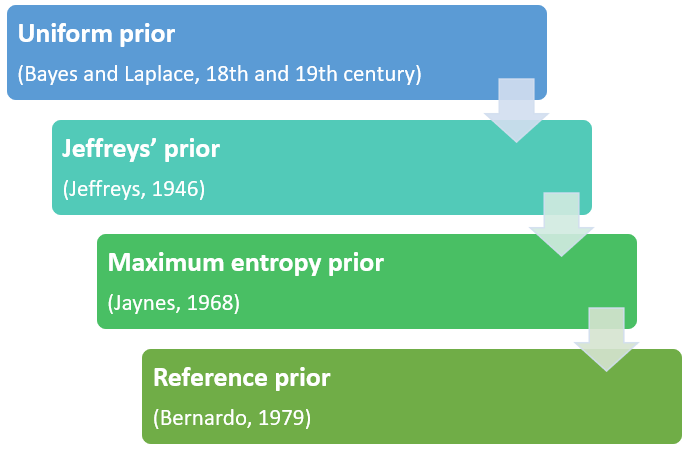## Prior versus evidence

In Bayesian inference, we use the data (or evidence) to update a prior.

As a result, we obtain a posterior distribution that combines the information contained in the prior with that coming from the data.

If the contribution of the prior is negligible with respect to that provided by the data, then we say that the prior is uninformative.

## Objective Bayesian statistics

Non-informative priors are the workhorse of objective Bayesian statistics.

In general, the prior reflects the statistician's subjective beliefs, as well as knowledge accumulated before observing the data.

However, there are many cases in which not only we have little prior knowledge, but we would also like not to rely on subjective beliefs.

This happens when scientific objectivity is at a premium (Lunn et al. 2013), for example, when:

• we aim to publish our analyses in a scientific journal;

• we are presenting results to a regulator or to another public body.

Uninformative priors are used to make Bayesian inferences as objective as possible.

## Criticism

There is a heated debate between so-called "objective" and "subjective" Bayesian statisticians (e.g., Berger 2006).

Uninformative priors are at the center of the debate.

Some statisticians think that the term uninformative is misleading and a misnomer because all priors contain some information.

Others (e.g. Berger 2006) argue that, even if uninformative priors are not really uninformative, the statistical community largely considers them "default" or "conventional" priors that can be used when an objective analysis needs to be performed. In Kass and Wasserman's (1996) words:

"There is no objective, unique prior that represents ignorance. Instead, reference priors are chosen by public agreement, much like units of length and weight. In this interpretation, reference priors are akin to a default option in a computer package. We fall back to the default when there is insufficient information to otherwise define the prior."

## Types of uninformative priors

We will briefly describe below the following classes of non-informative priors:

• Bayes-Laplace uniform prior;

• Jeffreys' prior;

• Jaynes' maximum entropy prior

• Bernardo's reference prior.

## Uniform prior

Since the earliest studies of Bayes and Laplace (see Fienberg 2006), there has been some agreement on the fact that one of the simplest ways to represent a state of ignorance about the value of a scalar parameteris to assign a uniform distribution to it.

With a uniform prior, each value ofhas the same prior probability (or probability density).

Denote the set of possible values of(its support) by.

Ifis finite and it haselements, then the prior probability mass is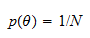for any.

Ifis a closed interval of length, then the prior probability density is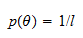for any.

In the other cases (e.g., infinite but countable support, or unbounded interval), the following improper uniform prior is often used: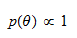In other words,is proportional to a constant.

An improper prior does not sum or integrate to. As such, it does not represent a proper probability distribution. However, in many interesting cases, it can be used, together with the likelihood of the observed data, as if it were a proper prior, to derive a proper posterior distribution.

## The problem of re-parametrization

What happens when we assign a uniform prior toand then we perform a re-parametrization, that is, we define a new parametersuch that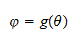whereis an invertible function?

It may happen thathas a non-uniform distribution.

Thus, the rule "always assign a uniform prior to the parameter" may produce inconsistent results. We may get two different priors forin the two cases in which

1. we directly assign a uniform prior to;

2. we first assign a uniform prior to, and then we compute the prior onby using the rules for calculating the probability distribution of a transformation.

## Jeffreys' prior

Jeffreys (1946) proposed a method to assign priors that is consistent under re-parametrization.

Suppose that the likelihood of a single observationisThen, Jeffreys' prior is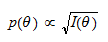whereis the Fisher information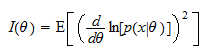Wikipedia has a detailed article on Jeffreys' prior, which shows some of its advantages:

• it is consistent under re-parametrization;

• it is often easy to derive analytically;

• in several important cases, it is the same as a uniform prior (possibly after performing a simple re-parametrization);

• it has a straightforward multivariate generalization: whenis a vector, the prior is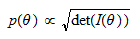whereis the Fisher information matrix.

However, Jeffreys' prior is often improper, and its multivariate version may have paradoxical properties (Dawid et al. 1973). These are the two main reasons why other priors have been proposed in the literature.

## Jaynes' principle of maximum entropy

Jaynes (1968) proposed another method to elicit uninformative priors.

He argued that the probability distribution which best represents a given state of knowledge can be found by maximizing the entropy of the distribution, subject to some constraints representing the available information.

Entropy is a measure of how "surprising" the realization of a random variable is, on average. The larger the entropy, the less information we have a priori.

If the supportof the parameter is finite, the entropy is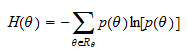Moreover, whenis finite and there are no constraints (no prior information), entropy is maximized by assigning a uniform prior to(i.e., all the possible values ofare equally likely).

Whenis not finite, we need to use a different definition of entropy (relative entropy) that requires us to define a base measure (often an arbitrary or subjective choice). The resulting prior can differ from a uniform prior.

The principle of maximum entropy has the advantage that it can be used to incorporate objective prior information by imposing constraints on the probability distribution of, without also introducing subjective views.

See the Wikipedia articles on the maximum entropy principle and maximum entropy probability distributions.

## Bernardo's reference prior

Bernardo (1979) introduced reference priors.

The starting idea is that the prior should influence the posterior as little as possible.

For this to happen, the posterior should be on average very different from the prior.

The difference between the posterior and the prior can be measured by the Kullback-Leibler divergence.

Therefore, a reference prior is derived by maximizing the expected value of the Kullback-Leibler divergence of the prior from the posterior.

In the univariate case, the reference prior turns out to be the same as Jeffreys' prior (e.g., Kaptein et al. 2022).

However, in the multivariate case, it often has better properties, as it does not generate the paradoxes generated by Jeffreys' prior.

The best way to learn about reference priors is to read the relevant chapter in the Handbook of Statistics (Bernardo 2005; freely available online).

## References

Berger, J., 2006. The case for objective Bayesian analysis. Bayesian analysis, 1(3), 385-402.

Bernardo, J.M., 1979. Reference posterior distributions for Bayesian inference. Journal of the Royal Statistical Society: Series B (Methodological), 41(2), 113-128.

Bernardo, J.M., 2005. Reference analysis. Handbook of statistics, 25, 17-90.

Dawid, A.P., Stone, M. and Zidek, J.V., 1973. Marginalization paradoxes in Bayesian and structural inference. Journal of the Royal Statistical Society: Series B (Methodological), 35(2), 189-213.

Fienberg, S.E., 2006. When did Bayesian inference become "Bayesian"? Bayesian analysis, 1(1), 1-40.

Jaynes, E.T., 1968. Prior probabilities. IEEE Transactions on systems science and cybernetics, 4(3), 227-241.

Jeffreys, H., 1946. An invariant form for the prior probability in estimation problems. Proceedings of the Royal Society of London. Series A. Mathematical and Physical Sciences, 186(1007), 453-461.

Kaptein, M. and van den Heuvel, E., 2022. Statistics for Data Scientists: An Introduction to Probability, Statistics, and Data Analysis. Springer Nature.

Kass, R.E. and Wasserman, L., 1996. The selection of prior distributions by formal rules. Journal of the American statistical Association, 91(435), pp.1343-1370.

Lunn, D., Jackson, C., Best, N., Thomas, A. and Spiegelhalter, D., 2013. The BUGS book. A Practical Introduction to Bayesian Analysis, Chapman Hall, London.